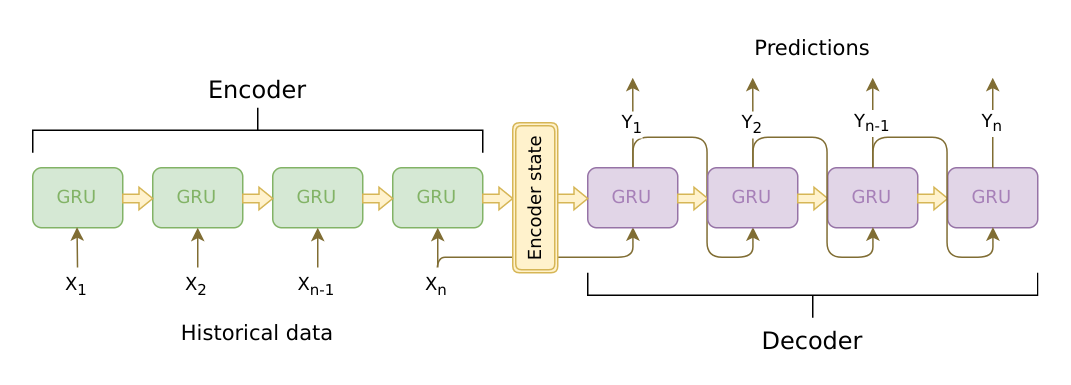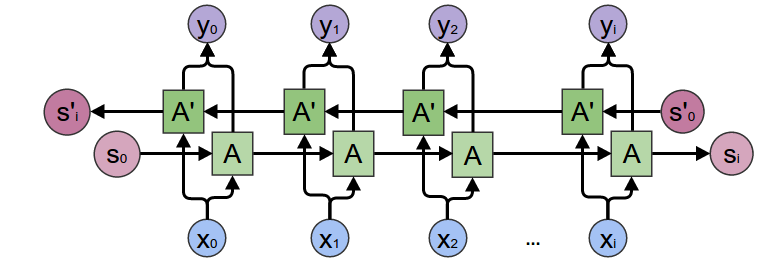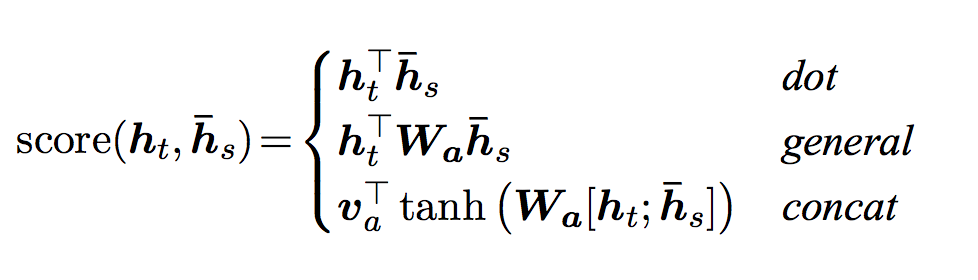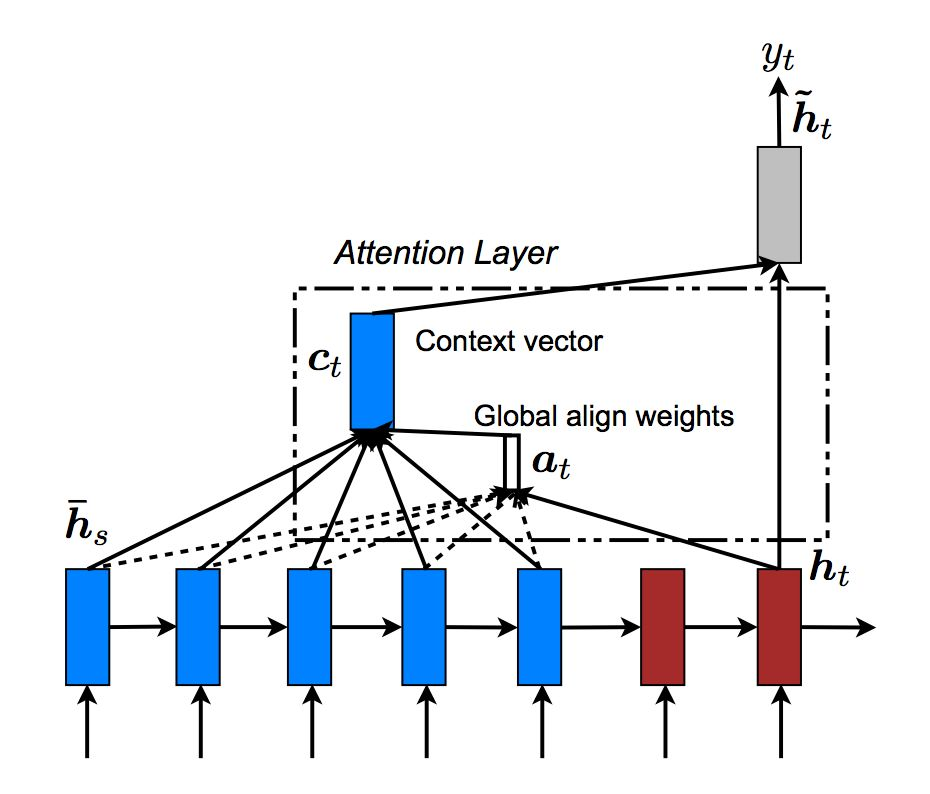一步步读懂Pytorch Chatbot Tutorial代码(五) – 定义模型 原创1779-孙同学

代码出处

Pytorch的CHATBOT TUTORIAL

https://pytorch.org/tutorials/beginner/chatbot_tutorial.html?highlight=gpu%20training

代码及说明 Define Models

Seq2Seq 模型Encoder

Encoder是个RNN，它会遍历输入的每一个Token(词)，每个时刻的输入是上一个时刻的隐状态和输入，然后会有一个输出和新的隐状态。这个新的隐状态会作为下一个时刻的输入隐状态。每个时刻都有一个输出，对于seq2seq模型来说，通常只保留最后一个时刻的隐状态，认为它编码了整个句子的语义，但是后面会用到Attention机制，它还会用到Encoder每个时刻的输出。Encoder处理结束后会把最后一个时刻的隐状态作为Decoder的初始隐状态。1. 把词的ID通过Embedding层变成向量。

3. 传入GRU进行Forward计算。

4. Unpack计算结果

5. 把双向GRU的结果向量加起来。

6. 返回(所有时刻的)输出和最后时刻的隐状态。

input_seq: 一个batch的输入句子，shape是(max_length, batch_size)

input_lengths: 一个长度为batch的list，表示句子的实际长度。

hidden: 初始化隐状态(通常是零)，shape是(n_layers x num_directions, batch_size, hidden_size)

outputs: 最后一层GRU的输出向量(双向的向量加在了一起)，shape(max_length, batch_size, hidden_size)

hidden: 最后一个时刻的隐状态，shape是(n_layers x num_directions, batch_size, hidden_size)

EncoderRNN代码如下：

class EncoderRNN(nn.Module):
def __init__(self, hidden_size, embedding, n_layers=1, dropout=0):
super(EncoderRNN, self).__init__()
self.n_layers = n_layers
self.hidden_size = hidden_size
self.embedding = embedding

# Initialize GRU; the input_size and hidden_size params are both set to 'hidden_size'
#   because our input size is a word embedding with number of features == hidden_size

self.gru = nn.GRU(hidden_size, hidden_size, n_layers,
dropout=(0 if n_layers == 1 else dropout), bidirectional=True)

def forward(self, input_seq, input_lengths, hidden=None):
# Convert word indexes to embeddings
embedded = self.embedding(input_seq)
# Pack padded batch of sequences for RNN module
# Forward pass through GRU
outputs, hidden = self.gru(packed, hidden)
# Sum bidirectional GRU outputs
outputs = outputs[:, :, :self.hidden_size] + outputs[:, : ,self.hidden_size:]
# Return output and final hidden state
return outputs, hidden

forward

• 使用outputs.view(seq_len, batch, num_directions, hidden_size)得到4维的向量。

• 其中第三维是方向，第四位是隐状态。

outputs, hidden = self.gru(packed, hidden)得到outputs为(max_length, batch, hidden*num_directions)

return outputs, hidden 返回最终的输出和最后时刻的隐状态。

Decoder

Decoder也是一个RNN，它每个时刻输出一个词。每个时刻的输入是上一个时刻的隐状态和上一个时刻的输出。一开始的隐状态是Encoder最后时刻的隐状态，输入是特殊的。然后使用RNN计算新的隐状态和输出第一个词，接着用新的隐状态和第一个词计算第二个词，…，直到遇到，结束输出。普通的RNN Decoder的问题是它只依赖与Encoder最后一个时刻的隐状态，虽然理论上这个隐状态(context向量)可以编码输入句子的语义，但是实际会比较困难。因此当输入句子很长的时候，效果会很长。

y

1

,

,

y

5

y_1,…,y_5

y1,,y5，那么我们可以用attention概率加权得到当前时刻的context向量

0.6

y

1

+

0.1

y

2

+

+

0.1

y

5

0.6y_1+0.1y_2+…+0.1y_5

0.6y1+0.1y2++0.1y5

h

t

h_t

ht表示t时刻的GRU输出的新的隐状态，我们可以认为

h

t

h_t

ht表示当前需要翻译的语义。通过计算

h

t

h_t

ht

y

1

,

,

y

n

y_1,…,y_n

y1,,yn的得分，如果

h

t

h_t

ht

y

1

y_1

y1的得分很高，那么我们可以认为当前主要翻译词

x

1

x_1

x1的语义。有很多中score函数的计算方法，如下图所示：h

t

h_t

ht表示t时刻的隐状态，比如第一种计算score的方法，直接计算

h

t

h_t

ht

h

s

h_s

hs的内积，内积越大，说明这两个向量越相似，因此注意力也更多的放到这个词上。第二种方法也类似，只是引入了一个可以学习的矩阵，我们可以认为它先对

h

t

h_t

ht做一个线性变换，然后在与

h

s

h_s

hs计算内积。而第三种方法把它们拼接起来然后用一个全连接网络来计算score。

h

t

h_t

ht

y

1

y_1

y1的内积、

h

t

h_t

ht

y

2

y_2

y2的内积，…。但是为了效率，可以一次计算

h

t

h_t

ht

h

s

=

[

y

1

,

y

2

,

,

y

n

]

h_s=[y_1,y_2,…,y_n]

hs=[y1,y2,,yn]的乘积。 计算过程如下图所示。dot_socre

encoder_outputs的shape是(input_lengths=10, batch=64, hidden_size=500)
hidden * encoder_output得到的shape是(10, 64, 500)，然后对第3维求和就可以计算出score。

forward

encoder_outputs的shape是(input_lengths=10, batch=64, hidden_size=500)

attn_energies = attn_energies.t()attn_energies从(max_length=10, batch=64)转置成(64, 10)

F.softmax(attn_energies, dim=1).unsqueeze(1)使用softmax函数把score变成概率，shape仍然是(64, 10)，然后用unsqueeze(1)变成 (64, 1, 10)

# Luong attention layer
class Attn(nn.Module):
def __init__(self, method, hidden_size):
super(Attn, self).__init__()
self.method = method
if self.method not in ['dot', 'general', 'concat']:
raise ValueError(self.method, "is not an appropriate attention method.")
self.hidden_size = hidden_size
if self.method == 'general':
self.attn = nn.Linear(self.hidden_size, hidden_size)
elif self.method == 'concat':
self.attn = nn.Linear(self.hidden_size * 2, hidden_size)
self.v = nn.Parameter(torch.FloatTensor(hidden_size))

def dot_score(self, hidden, encoder_output):

def general_score(self, hidden, encoder_output):
energy = self.attn(encoder_output)

def concat_score(self, hidden, encoder_output):
energy = self.attn(torch.cat((hidden.expand(encoder_output.size(0), -1, -1), encoder_output), 2)).tanh()

def forward(self, hidden, encoder_outputs):
# Calculate the attention weights (energies) based on the given method
if self.method == 'general':
attn_energies = self.general_score(hidden, encoder_outputs)
elif self.method == 'concat':
attn_energies = self.concat_score(hidden, encoder_outputs)
elif self.method == 'dot':
attn_energies = self.dot_score(hidden, encoder_outputs)

# Transpose max_length and batch_size dimensions
attn_energies = attn_energies.t()

# Return the softmax normalized probability scores (with added dimension)
return F.softmax(attn_energies, dim=1).unsqueeze(1)

1. 把词ID输入Embedding层

2. 使用单向的GRU继续Forward进行一个时刻的计算。

3. 使用新的隐状态计算注意力权重

4. 用注意力权重得到context向量

5. context向量和GRU的输出拼接起来，然后再进过一个全连接网络，使得输出大小仍然是hidden_size

6. 使用一个投影矩阵把输出从hidden_size变成词典大小，然后用softmax变成概率 7) 返回输出和新的隐状态

input_step: shape是(1, batch_size)

last_hidden: 上一个时刻的隐状态， shape是(n_layers x num_directions, batch_size, hidden_size)

encoder_outputs: encoder的输出， shape是(max_length, batch_size, hidden_size)

output: 当前时刻输出每个词的概率，shape是(batch_size, voc.num_words)

hidden: 新的隐状态，shape是(n_layers x num_directions, batch_size, hidden_size)

class LuongAttnDecoderRNN(nn.Module):
def __init__(self, attn_model, embedding, hidden_size, output_size, n_layers=1, dropout=0.1):
super(LuongAttnDecoderRNN, self).__init__()
# 保存到self里，attn_model就是前面定义的Attn类的对象。
# Keep for reference
self.attn_model = attn_model
self.hidden_size = hidden_size
self.output_size = output_size
self.n_layers = n_layers
self.dropout = dropout

# Define layers
# 定义Decoder的layers
self.embedding = embedding
self.embedding_dropout = nn.Dropout(dropout)
self.gru = nn.GRU(hidden_size, hidden_size, n_layers, dropout=(0 if n_layers == 1 else dropout))
self.concat = nn.Linear(hidden_size * 2, hidden_size)
self.out = nn.Linear(hidden_size, output_size)

self.attn = Attn(attn_model, hidden_size)

def forward(self, input_step, last_hidden, encoder_outputs):
# 注意：decoder每一步只能处理一个时刻的数据，因为t时刻计算完了才能计算t+1时刻。
# input_step的shape是(1, 64)，64是batch，1是当前输入的词ID(来自上一个时刻的输出)
# 通过embedding层变成(1, 64, 500)，然后进行dropout，shape不变。
# Note: we run this one step (word) at a time
# Get embedding of current input word
embedded = self.embedding(input_step)
embedded = self.embedding_dropout(embedded)
# 把embedded传入GRU进行forward计算
# 得到rnn_output的shape是(1, 64, 500)
# hidden是(2, 64, 500)，因为是双向的GRU，所以第一维是2。
# Forward through unidirectional GRU
rnn_output, hidden = self.gru(embedded, last_hidden)
# 计算注意力权重， 根据前面的分析，attn_weights的shape是(64, 1, 10)
# Calculate attention weights from the current GRU output
attn_weights = self.attn(rnn_output, encoder_outputs)
# encoder_outputs是(10, 64, 500)
# encoder_outputs.transpose(0, 1)后的shape是(64, 10, 500)
# attn_weights.bmm后是(64, 1, 500)

# bmm是批量的矩阵乘法，第一维是batch，我们可以把attn_weights看成64个(1,10)的矩阵
# 把encoder_outputs.transpose(0, 1)看成64个(10, 500)的矩阵
# 那么bmm就是64个(1, 10)矩阵 x (10, 500)矩阵，最终得到(64, 1, 500)
# Multiply attention weights to encoder outputs to get new "weighted sum" context vector
context = attn_weights.bmm(encoder_outputs.transpose(0, 1))
# 把context向量和GRU的输出拼接起来
# rnn_output从(1, 64, 500)变成(64, 500)
# Concatenate weighted context vector and GRU output using Luong eq. 5
rnn_output = rnn_output.squeeze(0)
# context从(64, 1, 500)变成(64, 500)
context = context.squeeze(1)
# 拼接得到(64, 1000)
concat_input = torch.cat((rnn_output, context), 1)
# self.concat是一个矩阵(1000, 500)，
# self.concat(concat_input)的输出是(64, 500)
# 然后用tanh把输出返回变成(-1,1)，concat_output的shape是(64, 500)
concat_output = torch.tanh(self.concat(concat_input))
# out是(500, 词典大小=7826)
# Predict next word using Luong eq. 6
output = self.out(concat_output)
# 用softmax变成概率，表示当前时刻输出每个词的概率。
output = F.softmax(output, dim=1)
# 返回 output和新的隐状态
# Return output and final hidden state
return output, hidden

评论 抢沙发觉得文章有用就打赏一下文章作者

支付宝扫一扫打赏微信扫一扫打赏Vieu3.3主题Q Q 登 录